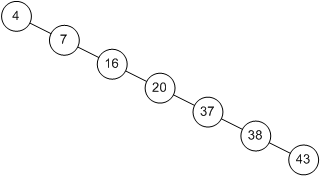Welcome to Lesson 10!

Learning Objectives
By the end of this class, you should know...
• How to analyze the run-time and algorithmic run-time of algorithms
• What is Big O notation?
• What are Binary and Linear Search and how do we analyze their run-times?
• If time: What is a Tree

Announcements

• Midterm 1 returned next class
• Project Report 2 due
• Submit Lab 4
• Lab 5 due next class
• Submit extra credit

Introduction to Run-Time Analysis

• Run-time analysis is used to determine the efficiency of an algorithm and estimate its run-time.
• For example, sorting a large array of numbers can take seconds, hours or even years depending on the algorithm used.
• However, we can not simply use a stop watch to compare algorithms.
• The physical run-time will change depending on the speed of the processor used.
• Consider implementing an algorithm in C++ and then running the program on a super computer vs your grandma's old desktop from the 1980s.
• Instead, we are concerned with how the run-time of an algorithm scales mathematically as its input size increases.
• In other words, how well will an algorithm work if given a 10 element array vs a 10,000 element array vs a 1 million element array?
• We can represent an algorithm as a mathematical function of the input size of the problem.
• For example, to estimate the efficiency of an algorithm, we might count the number of steps in the algorithm.
• However, the number of steps in the algorithm is also dependent on the size of the problem.
• The algorithm can be carried out faster on a smaller problem and slower on a larger one.
• We can label the size of the problem n, where n is a variable to represent any problem size.
• After we calculate the steps in an algorithm, we can write what is called it's "run-time equation", which is a function written in terms of n.
• For example, a run-time equation might look like this: 4n2 + 2n + 1
• Once we know the run-time equation of an algorithm, we can use a mathematical metric for run-time ("Big-O") to compare algorithms independent of hardware.
• The algorithm above has a "Big-O" run-time of O(n2)
• We can compare this algorithm to other algorithms by comparing their "Big-O" run-times.• Space efficiency can also be measured this way, and may also be important, depending on resource constraints.
• In computer science we are less concerned with space efficiency.

Types of Run-Time Analysis

• When analyzing the run-time of an algorithm, we do so theoretically (remember, we are not interested in the performance on any specific machine).
• Specifically, we want to consider three possibilities:
• Best case - Given an input, the algorithm requires the least amount of computation, i.e. runs the fastest.
• Worst case - Given an input, the algorithm requires the most amount of computation, i.e. runs the slowest.
• Average case - Given an average case random input, provides a prediction about the average amount of computation, i.e. the average run-time.
• These are usually expressed as an order of magnitude in terms of "Big-O".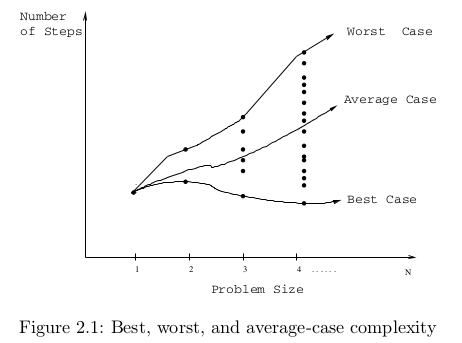For Example:

• Let's consider the algorithm linear search, which searches an array, index-by-index, to find a specific value.
• If the item is in the array, we return the location index; otherwise, we return -1 (an impossible array index).
• Recall the code for linear search looks like this:
//Pre: array not empty
int linearSearch(int array[], int valueToFind, const int SIZE)
{
for(int i = 0; i < SIZE; i++)
{
if (array[i] == valueToFind)
{
return i;
}
}
return -1;
}
• Let's consider the best case run-time for this algorithm. What if the array has 100 elements in it? What input would cause this algorithm to run for the least amount of time? (I am only looking for a verbal description here).
• What about the worst case?
• And the average case (what happens most of the time)?

Orders of Growth: Big-O Notation

• Big-O Notation is the mathematical formalism used in computer science to express how the run-time of an algorithm scales with input.
• We write Big-O notation, like so:

O(growth rate function)

• The growth rate function represents the leading term of the run-time equation. The leading term in a function is the term which has the greatest impact on the run-time of the algorithm.
• We typically express the growth rate function as a function of the input size, n. For example:
O(n) or O(n2) or O(log(n))
• The capital O stands for "of order".
• You can think of order here meaning of "the same general size."
• For example: A = O(n) means that the algorithm A is of order n.
• We could have another algorithm B = O(n).
• These are two different algorithms, but they have the same "order."
• In other words, they are considered to be equally efficient.
• We could also have a third algorithm C = O(n2).
• This is a different algorithm with a different order.
• Algorithm C would be considered less efficient.
• The big O of A, B and C tells us that A and B grow at a very similar rate as input size increases, but that C grows faster (and will take longer to run).
Linear Search Example
• Given our example above about linear search, we can express the best, worst and average case run-times using big O notation:

Best case:          1        ->          O(1)  // We found the item on the first try.

Worst case:        n       ->           O(n) // We have to search all n nodes in the list to find the item.

Average case:      n/2   ->           O(n) // We have to search an average of half of the n nodes in the list to find the item.

But wait! Shouldn't that be O(n/2)?

• Note that when we express the order of magnitude, we drop any lesser terms and coefficients on our variables.
• These lesser terms and coefficients are considered insignificant in comparison to the growth rate function (the highest order term).
• The lesser terms and coefficients have little impact on the overall efficiency of the algorithm.
• So, for the average case above, you might think you should express it as O(n/2). However, since we drop coefficients, we write it simply as O(n).
Another Example
• As another example, if we know the the run-time of a specific algorithm in its worst case is:
f(n) = 3n4 + 100n2 + 10n + 50

We would express its order of magnitude as O(n4).

f(n) = 3n4 + 100n2 + 10n + 50 = O(n4)

Note the use of the equals sign above.

Some Common Orders of Magnitude
• O(1) is called bounded or constant time. The amount of work is bounded by a constant and does not depend on the size of the problem.
• For example: Assigning a value to a single index of an array is O(1).

• O(log2 n) is called logarithmic time. The amount of work depends on the log of the size of the problem. Algorithms that successively cut the amount of data to be processed in half typically fall into this category.
• For example: Binary search (as we shall discuss in a moment) and other "Divide and Conquer" algorithms.

• O(n) is called linear time. The amount of work is typically some constant times the size of the problem.
• For example: Printing each element in a list of n elements. Or, searching a list of unordered elements, as we saw in the linear search example above.

• O(nlog2 n) is called (for lack of a better term) nlog(n) time. Algorithms of this type typically involve applying a logarithmic time algorithm n times - such as applying a logarithmic algorithm to all n items in a list.
• For example: The better sorting algorithms such as Quicksort, Heapsort and Mergesort.

• O(n2) is called quadratic time. Algorithms of this type typically involve applying a linear algorithm n times. They also typically have a nested loop structure, such as a nested for loop.
• For example, most simple sorting algorithms are O(n2).

• O(2n) is called exponential time. These algorithms are costly, as the exponential times increase dramatically in relation to the size of n. They should only be used on very small data sets or not at all.
• For example, our recursive fibonacci function from the previous class is approximately O(2n), since for each of the n inputs, we broke the problem into two recursive calls.

• O(n!) is called factorial time. These algorithms are even more costly and can involve what is called "brute force" solutions (rather than efficient, well-thought-out solutions).
• For example, a brute-force solution to the Traveling Salesman Problem (which you will probably learn more about in Discrete Math).

Graphical Comparison of Common Orders of Magnitude

Numerical Comparison of Rates of Growth

 Input 1 log n n nlogn n2 2n 1 1 0 1 0 1 2 2 1 1 2 2 4 4 4 1 2 4 8 16 16 8 1 3 8 24 64 256 16 1 4 16 64 256 65,536 32 1 5 32 160 1,024 4,294,967,296 64 1 6 64 384 4,096 About a month of instructions on a super-computer 128 1 7 128 896 16,384 About 1012 times greater than the age of the universe in nanoseconds 256 1 8 256 2,048 65,536 Don't ask

Review of Linear Search Run-Time

• We saw when we analyzed Linear Search that it add the following run-times:
• Best Case: O(1)
• Average Case: O(n)
• Worst Case: O(n)
• Thus, we consider Linear Search to be an O(n) algorithm.
• A brief examination of its code reveals confirms it as an O(n) algorithm because it has a for loop that goes through all n elements in the array.
bool linearSearch(int array[], int value, const int SIZE)
{
for(int i = 0; i < SIZE; i++) <-- single loop generally indicates an O(n)algorithm
{
if (array[i] == value)
{
return true;
}
}
return false;
}

Binary Search Run-Time: Worst Case
• But, what about a search algorithm that we know to be faster: binary search?
• How can we analyze its best, worst and average case run-times?

• How many times did we have to split the array in half before it "became empty"?
• It took us 4 passes.
• In general, we can split an array in half floor(log2(n)) + 1 times before it becomes empty.
• Recall that logbase value = exp is equivalent to baseexp = value
•  Or, logb a = c is equivalent to bc = a.
• In our example with 15 elements, we needed 4 comparisons in the worst case.
• floor(log2(15)) + 1 = 3 + 1 = 4 <--The formula works!
• Therefore, Binary Search is O(log2 n) in the worst case. (Remember that we drop the + 1).
• For algorithms that are log2 n, each time you double the number the number of elements in the array or other structure, the amount of work you do increases by just one unit.Binary Search Pays Off!

Number of Elements             Number of Comparisons
_______________________________________________________
15                                           4
31                                           5
63                                           6
127                                         7
255                                         8
511                                         9
1023                                       10
1 million                               20
• This is a very significant savings over linear search. However, binary search requires a sorted array , whereas linear search does not. If we need to sort the array before applying the binary search algorithm, there will be a loss of efficiency.

Binary Search Average and Best Case
• The average case of binary search is also O(log2 n) as it takes on average one fewer comparison than in the worst case.
• What is the best case?

Linear vs Binary Search

Analysis of Algorithms Practice
Find a partner and discuss the following: What is the Big-O run-time of the below functions?

A.
void List:print()
{
while (temp != NULL)
{
cout << temp->data << " ";
temp = temp->next;
}
cout << endl;
}

B.

void bubble_sort(int arr[], int n)
{
for (int i = 0; i < n; ++i)
{
for (int j = 0; j < n - i - 1; ++j)
{
if (arr[j] > arr[j + 1])
{
int temp = arr[j];
arr[j] = arr[j + 1];
arr[j + 1] = temp;
}
}
}
cout << "The array contents are:"<< endl;
for(int k = 0; k < n; k++)
{
count << "Element " << k << " is " << arr[k] << endl;
}
}

C.
void mysteryFunction(int n)
{
int i = 0;
while (i < n)
{
for (int j = 0; j < n; j++)
{
for (int k = 0; k < n/2; k++)
{
if (j < k && k > i)
cout << "*";
}
cout << endl;
}
i++
}
}

Introducing Trees
• Consider the ADTs we have studied so far:
• Array
• Queue
• Stack
• These structures all store data in a linear fashion, as in the example of a linked list show below:• However, storing data linearly is not the only option.
• In many cases, our data might not have a linear relationship.
• Consider, for example, a company's org. chart:• Or, consider this depiction of the relationship among the countries in the world and their cities and states:Image source.

• Or, consider this data which is arranged into what we call a decision tree: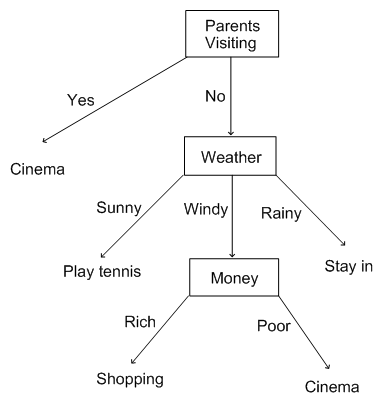• Given the nature of certain types of data, sometimes it can make more sense to organize the data in hierarchical structure.
• Also, certain operations, such as search and insertion or deletion can be more efficient when we organize data hierarchically rather than linearly.
• In computer science, we can use a tree ADT to organize data hierarchically.

The Structure of the Tree ADT
• Like linked lists, trees are comprised of nodes.
• These nodes store data and pointers to other nodes in the tree.
• Like the head and tail of a linked list, trees have specially-named nodes that serve important functions in the tree.
• The most important node is known as the root.
• Like the head of a linked list, a root is what allows us to access the first node in the tree.
• You can visualize a tree ADT with its root node like this:• Trees in computer science are usually depicted with the root node on top.
• Therefore, you have to visualize the tree upside down.• The branches of the tree are called links or edges.
• Unless the tree is empty or has size==1, each Node in the tree is connected to other nodes by these pointers (links).
• Nodes in a tree have parent-child relationships.
• Each node can have only one parent node. But a node can have 0 or more child nodes.
• The root node in the image below has four child nodes.
• The root is considered the parent node of those four child nodes.
• For example, A is considered the child node of the root and the root is considered the parent node of A.• In other words, the terminology we use to describe trees and their nodes, is similar to that of a family tree.• Nodes in a tree can have parents, grandparents, cousins, siblings, children and grandchildren.
• They can also have ancestors and descendants.
• For example, in the tree below, Nodes 1,4,7, and 10 and their parents are all descendants of the the root node 5.
• The root node 5 is thus their ancestor.• Another important node in a tree is the leaf node.
• The leaf node is any node in the tree with no children.
• In other words, the links of a leaf node point to Null.
• Any node that is not the root or a leaf is called an interior node as depicted above.
• The root and leaves of the tree are exterior nodes.

Properties of Trees
• Consider a tree with n nodes. How many edges will it contain.
• Exactly n-1. Why is this the case?
• The reason is that all nodes, except the root, have exactly one incoming edge.
• The depth of a node X in a tree is defined as the number of edges from the root to the node X.
• What is the depth of the root node, given this definition?
• The height of a node X is defined as the number of edges in the longest path from the node X down to a leaf node.
• What is the height of any leaf node given this definition?
• Trees themselves can also have heights. The height of any tree is the same as the height of its root.Activity Labeling a Tree
• Given the tree below, answer the following questions:• Step 1: Label the root and leaf nodes
• Step 2: List at least one of each of the following relationships:
• Parent-child
• Child-parent
• Siblings
• Cousins
• Grandparent-grandchild
• Ancestor
• Descendant
• Step 3: identify 3 interior nodes
• Step 4: label the depth of the following nodes:
• /
• -
• f
• g
• 2 (both of them)
• Step 5: label the height of the same nodes.
• A binary tree is a tree where each node can have at most 2 children.
• Below is an example of a binary tree. Note that each node has 0, 1 or 2 children.Image source
• These two children are called the left child and the right child.
• For example, in the BT above, 4 is the left child of its parent node 6 and 7 is the right child of its parent node 6.
• 14 only has a left child, but does not have a right child.
• We can consider binary trees to be recursive structures.
• The root node links to root nodes of its left and right subtrees.
• These roots of the subtrees in turn link to the root nodes of their left and right subtrees.
• We will discuss this concept more when we get to Binary Search Trees.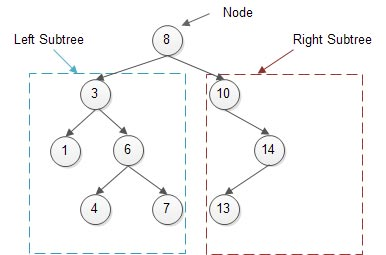• Therefore, each node in the tree will contain some data as well as two pointers, one to the left child and one to the right child as depicted below:• If a node has no right or left child, these values are set to Null.
• As you might have predicted, when we go to implement the binary search tree, we will be representing its node as a private inner struct like this:
struct Node
{
int data;
Node* leftchild;
Node* rightchild;
};
• Therefore, a Node has three fields:
• a field for the data
• a field for the address of the left child (another Node).
• a field for the address of the right child (another Node).
• A binary tree is made up of Nodes, each with pointers to a left and right child node as shown below: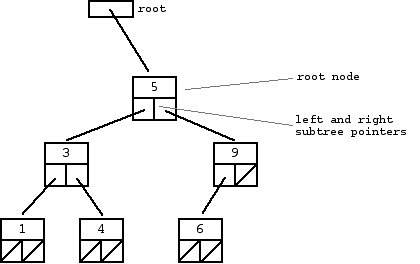• The examples of binary trees that we have seen so far are balanced trees.
• Here is another example of a valid binary tree. Why does this tree meet the definition of a binary tree?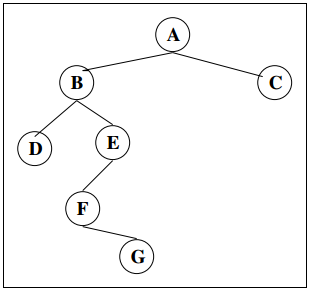• This is also a valid binary tree. Why? Also, what does it resemble?